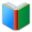# List 1 for 6th Grade MATH

Middle School Words: List 1 for 6th Grade MATH, random words are selected for the Word Quiz worksheet. This sheet is created dynamically, every sheet is different from previous ones. You can download and print the worksheet from browser directly.
 Actions upon current list

 All lists of current grdae
Spelling Words:

Arts Words:

Social Words:

Math Words:

Science Words:

Literature Words:The SwineherdThe Red ShoesA Study In Scarlet
 Worksheet - Middle School Words: List 1 for 6th Grade MATH

 Print: List 1 for 6th Grade MATH

Exam Word - https://www.examword.com/

Middle School Words: List 1 for 6th Grade MATH

 1: right line drawn or extending from the center of a circle to the periphery evaluate[__] similarity[__] prime factor[__] radius[__] 2: the resolution of an entity into factors such that when multiplied together they give the original entity sequences[__] factorization[__] radius[__] numerical expression[__] 3: the prime factors of a quantity are all of the prime quantities that will exactly divide the given quantity prime factor[__] square units[__] factorization[__] convert[__] 4: the action of following in order; serial arrangement in which things follow in logical order or a recurrent pattern convert[__] sequences[__] numerical expression[__] reciprocal[__] 5: boundary line of a circle, figure, area, or object pi[__] circumference[__] similarity[__] coordinate plane[__]